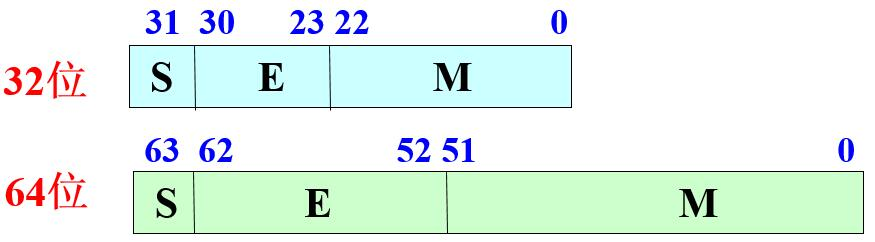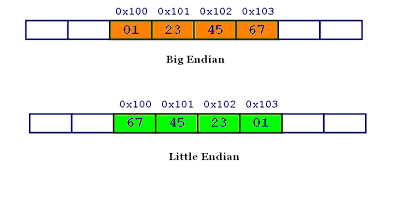# 标准

FP32 1 8 23
FP16 1 5 10
bFP16 1 8 7# 规格化表示Normalized

1.23 × 10^2^，0.123 × 10^3^，12.3 × 10^1^

(1) 尾数用原码,且隐藏尾数最高位。

(2) 阶码使用“移码”，基固定为2x=(−1)^s^×(1.M)×2^E−127^ 一个规格化的64位浮点数ｘ的真值为：

x=(−1)^s^×(1.M)×2^E−1023^

# 大端小端

• 大端字节序：高位字节在前，低位字节在后，这是人类读写数值的方法。
• 小端字节序：低位字节在前，高位字节在后，即以0x1122形式储存。

0x1234567的大端字节序和小端字节序的写法如下图。## Examples

(1) 首先转化为2进制表示

−3.75=−(2 + 1 + 1/2 + 1/4)=−1.111×2^1^ (2) 整理符号位并进行规格化表示

−1.111×2^1^=(−1)^(1)^ × (1+0.1110 0000 0000 0000 0000 000)×2^1^

(3) 进行阶码的移码处理 (−1)^(1)^×(1+0.1110 0000 0000 0000 0000 000)×2^1^ =(−1)^(1)^×(1+0.1110 0000 0000 0000 0000 000)×2^128−127^

1 1110 0000 0000 0000 0000 000 1000 0000